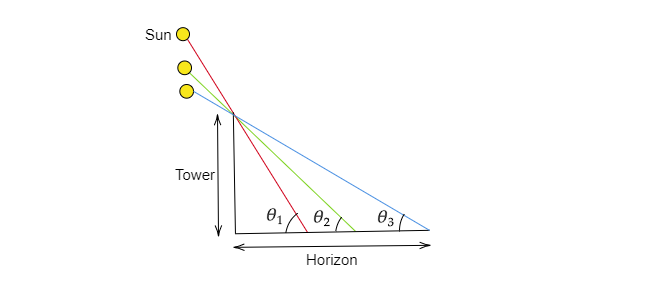# Write 'True' or 'False' and justify your answer in each of the following:If the length of the shadow of a tower is increasing, then the angle of elevation of the sun is also increasing.

Given:

If the length of the shadow of a tower is increasing, then the angle of elevation of the sun is also increasing.

To do:

We have to find whether the given statement is true or false.

Solution:We can express the angle of elevation as $tan\theta=\frac{height of the tower}{length of the shadow}$

$\therefore tan\theta$ get decreased as the the length of the shadow increases i.e $\theta$ get decreased.

In figure it has been shown that how the angle of elevation is decreasing with the increment in the length of the shadow.